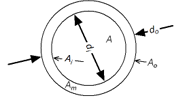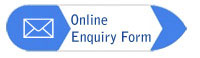# Pipe Weight Formula In Kg | Plate Weight Formula Metric | Sheet Weight Formula | Tube Weight Formula | Aluminium Weight Formula Kg | Flat Bar Weight Calculator

## Stainless Steel Sheet Weight Calculation Formula1. Formula for Calculating S. S. Pipe Weight :

0.D. (mm) - W. Thick (mm) X W. Thick (mm) X 0.0248 = Wt. Per Mtr.
0.D. (mm) - W. Thick (mm) X W. Thick (mm) X 0.00756 = Wt. Per Feet

##### Formula for calculating SS Pipe Weight

2. Formula for Calculating S. S. Bar Weight :
DIA (mm) X DIA (mm) X 0.00623 = Wt. Per Mtr.
DIA (mm) X DIA (mm) X 0.0019 = Wt. Per Feet
##### Formula for calculating SS Bar Weight

3. Formula for Calculating S. S. Square Bar Weight :
DIA (mm) X DIA (mm) X 0.00788 = Wt. Per Mtr.
DIA (mm) X DIA (mm) X 0.0024 = Wt. Per Feet
##### Formula for calculating SS Square Weight

4. Formula for Calculating S. S. Hexagonal Bar Weight:
DIA (mm) X DIA (mm) X 0.00680 = Wt. Per Mtr.
DIA (mm) X DIA (mm) X 0.002072 = Wt. Per Feet
##### Formula for calculating SS Hexagonal Weight

5. Formula for Calculating S.S. Flat Bar Weight:
Width (mm) X Thick (mm) X 0.00798 = Wt. Per Mtr.
Width (mm) X Thick (mm) X 0.00243 = Wt. Per Feet
##### Formula for calculating SS Flat Bar Weight

6. Formula for Calculating S.S. Sheet & Plates Weight:
Length (mtrs) X Width (mtrs) X Thick (mm) X 8.068 = Wt. Per PC.
Length (feet) X Width (feet) X Thick (mm) X 3/4 = Wt. Per PC
##### Formula for calculating SS Sheet & Plates Weight

7. Formula for Calculating S.S. Circle Weight:
Dia (mm) X Dia (mm) X Thick (mm) ÷ 160 = Gms. Per PC
Dia (mm) X Dia (mm) X Thick (mm) X 0.0000063 = Wt. Per PC
##### Formula for calculating SS Pipe Weight

8. Formula for Calculating Brass Pipe/ Copper Pipe Weight:
O. D. (mm) - Thick (mm) X Thick (mm) X 0.0260 = Wt. Per Mtr.
##### Formula for calculating SS Pipe Weight

9. Formula for Calculating Lead Pipe Weight:

O. D. (mm) - Thick (mm) X Thick (mm) X 0.0345 = Wt. Per Mtr.

##### Formula for calculating Lead Pipe Weight

10. Formula for Calculating Aluminium Pipe Weight:
O. D. (mm) - Thick (mm) X Thick (mm) X 0.008 = Wt. Per Mtr.
##### Formula for calculating Aluminium Pipe Weight

11. Formula for Calculating Aluminium Sheet Weight:
Length (Mtr.) X Width (Mtr.) X Thick (mm) 2.69 = Wt. Per PC
##### Formula for calculating SS Pipe Weight

12. Conversion of Mtrs to Feet:
Weight of 1 Mtrs ÷  3.2808  = Weight Per Feet
13. Bar Low's Formula for Calculating Bursting Pressure:
P = 2ST/D or t-DP/25S or S-DP/2t or D = 2st /P
P = Bursting Pressure Psi.
S = Tensile Strength of Tube
T = Wall Thickness (in inches)
D = Outside Diameter (in inches)

We Export to: We Import to:
People Also Search :

#### Pipe Weight Formula In Kg | Plate Weight Formula Metric | Sheet Weight Formula | Tube Weight Formula | Aluminium Weight Formula Kg | Flat Bar Weight Calculator

##### Stainless Steel Sheet Weight Calculator, SS Pipe Weight Calculator, SS Bar Weight Calculator, SS Square Bar Weight Calculator, SS Hexagonal Bar Weight Calculator, SS Flat Bar Weight Calculator
###### SS Sheet & Plates Weight Calculator, SS Circle Weight Calculator, Brass Pipe/ Copper Pipe Weight Calculator, Lead Pipe Weight Calculator, Aluminium Pipe Weight Calculator, Aluminium Sheet Weight Calculator, STEEL PIPE WEIGHT CALCULATOR, STAINLESS STEEL PIPE WEIGHT CALCULATOR